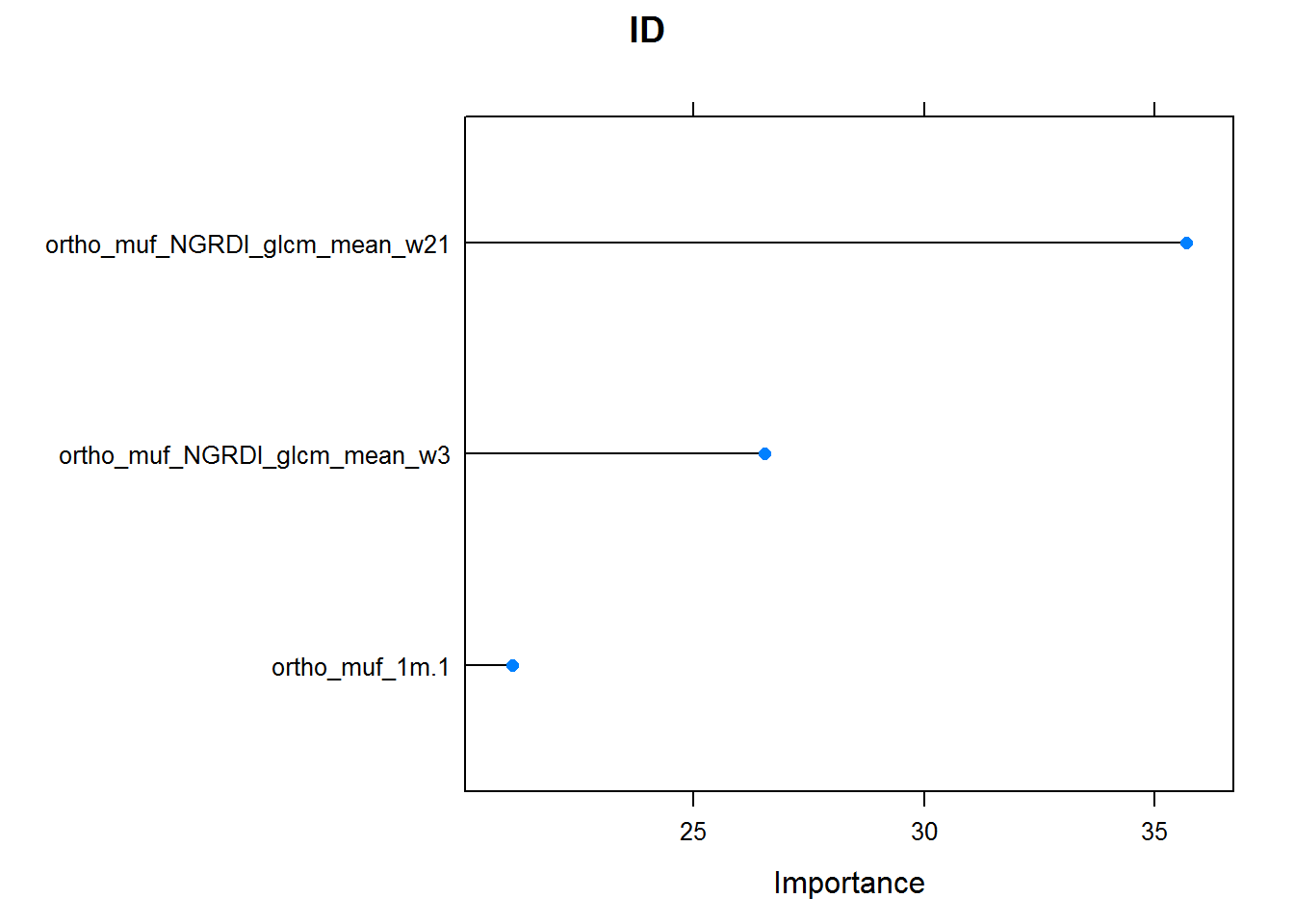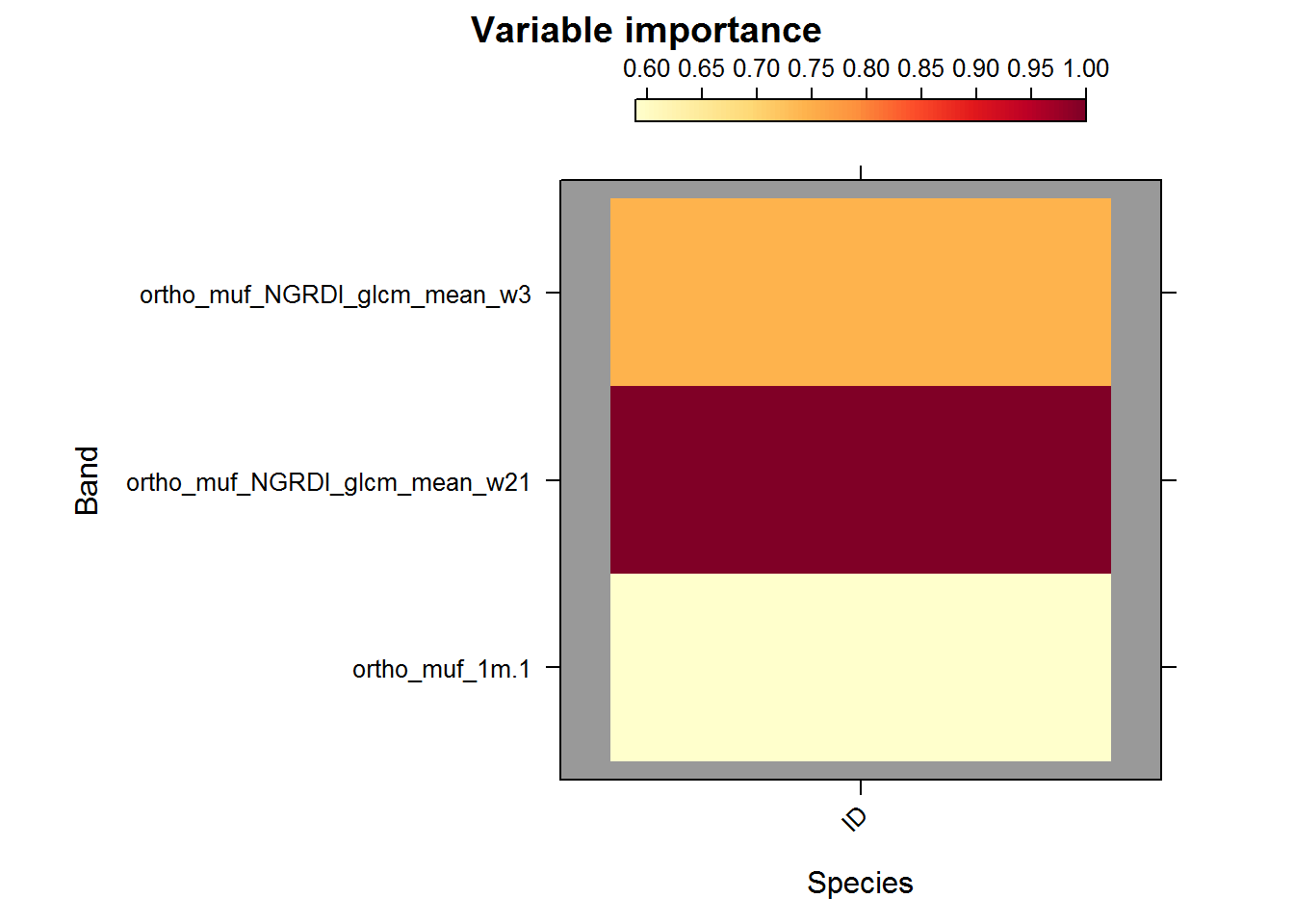#### Site Tools

courses:msc:msc-phygeo-remote-sensing:code-examples:rs-ce-07-1

# E07-1: Land-cover classification with R

The following examples illustrate the preprocessing of spatial data sets using the sp and raster package.

As always, we start with importing the packages and setting the working directory.

Before we can build a model, we need a comprehensive dataset which has been compiled by extracting the values of the raster layers used for the land-cover prediction from inside the training areas. Since this has already been done during previous worksteps, the tabulated (data.frame) information which relates landcover and the pixel values of all the raster layers in each row is read from an rds object. Based on this dataset, a new datasest of class gpm is created later. The column “ID” which holds the ID of the land-cover classes is transformed to a factor since otherwise, the machine learning models would run in regression mode (i.e. predict numeric values including decimal values and not classes).

obsv <- readRDS(file = paste0(path_muf_set1m_lcc_ta, "muf_lc_ta_poly_smpl_cmb_cc.rds"))
obsv <- obsv[which(obsv$ID %in% names(table(obsv$ID)[table(obsv$ID) > 100])),] obsv <- obsv[, c(which("ID" == colnames(obsv)), which("ortho_muf_1m.1" == colnames(obsv)), which("ortho_muf_NGRDI_glcm_mean_w3" == colnames(obsv)), which("ortho_muf_NGRDI_glcm_mean_w21" == colnames(obsv)))] obsv <- obsv[complete.cases(obsv),] obsv <- obsv[!is.infinite(obsv$ortho_muf_NGRDI_glcm_mean_w3),]
obsv <- obsv[!is.infinite(obsv$ortho_muf_NGRDI_glcm_mean_w21),] obsv$ID <- as.factor(obsv$ID) In order to build the gpm object, some meta information has to be defined. This mainly holds information on which column number to use as response (i.e. dependent) variable and which ones to use as independent variables. col_selector <- which(names(obsv) == "ID") col_meta <- NULL col_lc <- which(names(obsv) == "ID") col_precitors <- seq(which(names(obsv) == "ortho_muf_1m.1"), which(names(obsv) == "ortho_muf_NGRDI_glcm_mean_w21")) meta <- createGPMMeta(obsv, type = "input", selector = col_selector, response = col_lc, predictor = col_precitors, meta = col_meta) obsv <- gpm(obsv, meta, scale = FALSE) Once the gpm object has been created, it can be used for some more data preparation and model training as well as evaluation. Before we start with resampling/validation procedures, we can have a look at the correlation of the independent variables. Sometimes, the number of variables can considerably be decreased if highly correlated variables are excluded from the data (except for one of them for each highly correlated group). Since we use only three predictor variables in this example, we will skip this step but show only some commented code which illustrates a predictor variable reduction using a correlation threshold value of 0.80. # muf_gpm <- cleanPredictors(x = muf_gpm, nzv = TRUE, # highcor = TRUE, cutoff = 0.80) Prior to training the model, we create some resamples of the dataset and split them into test and training datasets afterwards. In the following, only 50 data pairs per land-cover are extracted from the overall dataset and this reampling procedure is repeaded ten times. Afterwards, each of the resamplings is split into a training and testing dataset (each holding 65 percent of the data). obsv <- resamplingsByVariable(x = obsv, use_selector = FALSE, resample = 5, grabs = 50) # Split resamples into training and testing samples obsv <- splitMultResp(x = obsv, p = 0.65, use_selector = FALSE) Once the resampling has been defined, the model tuning and feature selection is handeld by the trainModel function. obsv <- trainModel(x = obsv, n_var = NULL, mthd = "rf", mode = "rfe", seed_nbr = 11, cv_nbr = 5, var_selection = "indv", filepath_tmp = NULL) Based on the 10 trained models (10 because 10 resamplings have been created above), the variable importance of based on each model can be computed in a scaled (i.e. rainging between 0 and 1) and unscaled manner. var_imp <- compVarImp(obsv@model$rf_rfe, scale = FALSE)
var_imp_scale <- compVarImp(obsv@model$rf_rfe, scale = TRUE) The statistics can further be used to create some variable importants plots. Please note that the heatmap only makes sense if more than one type of response variable has been selected (e.g. if one predicts different classes separately and not within one model). plotVarImp(var_imp) ## []plotVarImpHeatmap(var_imp_scale, xlab = "Species", ylab = "Band")Finally, the performance statistics can be computed for regression or - as in this example - classification models. tstat <- compContTests(obsv@model$rf_rfe, mean = TRUE)
tstat[]["Kappa_mean"]
##   Kappa_mean
## 1   0.461751